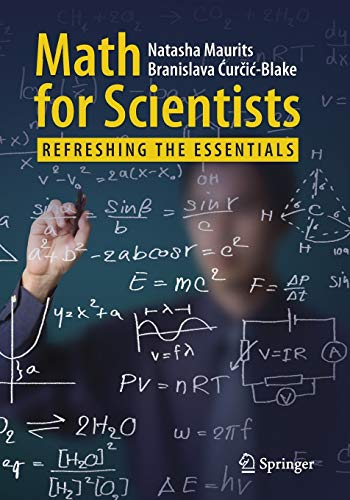# Math for Scientists: Refreshing the Essentials

• Length: 246 pages
• Edition: 1
• Publisher:
• Publication Date: 2017-09-07
• ISBN-10: 3319573535
• ISBN-13: 9783319573533
5.0
Description

This book reviews math topics relevant to non-mathematics students and scientists, but which they may not have seen or studied for a while. These math issues can range from reading mathematical symbols, to using complex numbers, dealing with equations involved in calculating medication equivalents, the General Linear Model (GLM) used in e.g. neuroimaging analysis, finding the minimum of a function, independent component analysis, or filtering approaches. Almost every student or scientist, will at some point run into mathematical formulas or ideas in scientific papers that may be hard to understand, given that formal math education may be some years ago. In this book we will explain the theory behind many of these mathematical ideas and expressions and provide readers with the tools to better understand them. We will revisit high school mathematics and extend and relate this to the mathematics you need to understand the math you may encounter in the course of your research. This book will help you understand the math and formulas in the scientific papers you read. To achieve this goal, each chapter mixes theory with practical pen-and-paper exercises such that you (re)gain experience with solving math problems yourself. Mnemonics will be taught whenever possible. To clarify the math and help readers apply it, each chapter provides real-world and scientific examples.